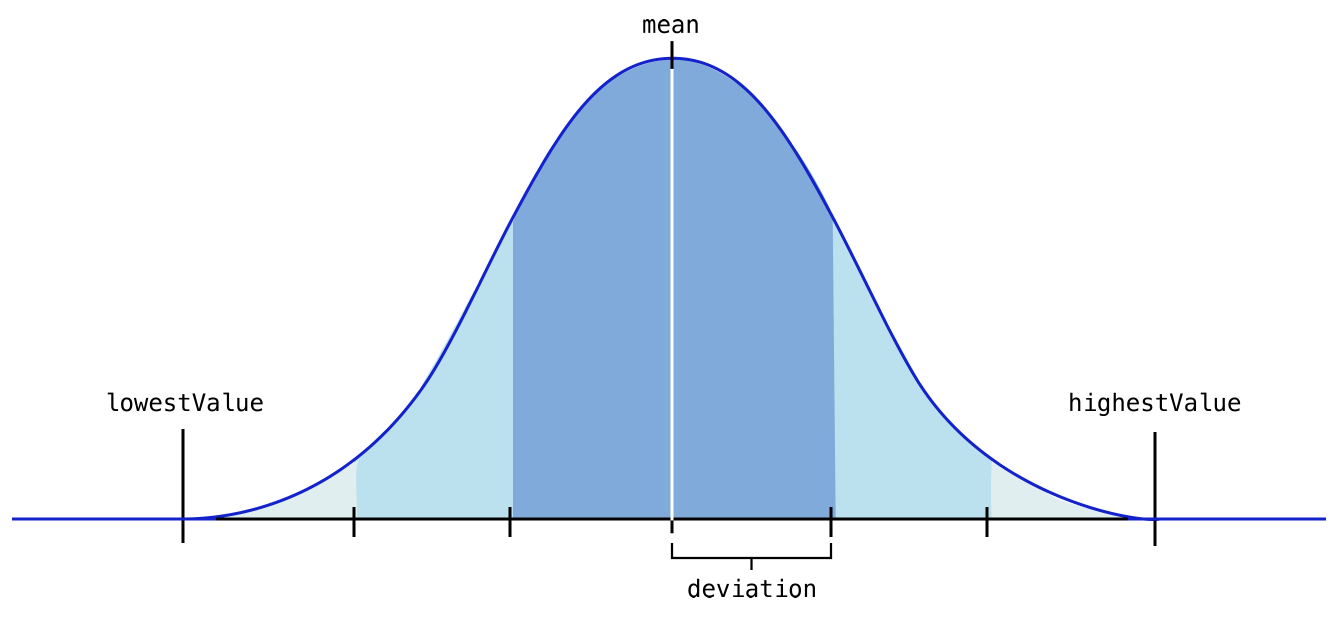Class

GKGaussianDistribution

A generator for random numbers that follow a Gaussian distribution (also known as a normal distribution) across multiple samplings.

OverviewFigure 1 Probabilities of Values Generated by a Gaussian Distribution

In a Gaussian distribution, random samplings are most likely to result in a value near the center of the distribution, and have reduced probability of producing higher or lower values. To generate random numbers with a Gaussian distribution, use the methods defined by its superclass GKRandomDistribution.

A Gaussian distribution is characterized by its mean and deviation properties. The mean value (also called the median or expected value) is the value at the center of the distribution (halfway between its lowestValue and highestValue properties), and the most likely value to result from a random sampling. The farther any other value is from the mean, the less likely that value is to result from a random sampling. The deviation value (also called standard deviation or sigma) characterizes those probabilities: 68.27% of values generated by the distribution are within one deviation of the mean, 95% of generated values are within two deviations, and 100% of generated values are within three deviations.

Gaussian distributions appear in many natural phenomena that you might model in a game. For example, the following code creates a distribution whose random number generation is equivalent to rolling three six-sided dice (also called 3d6) and summing the results.

For more information on choosing and using randomizers in GameplayKit, read Randomization in GameplayKit Programming Guide.

Topics

Creating a Random Distribution

init(randomSource: GKRandom, lowestValue: Int, highestValue: Int)

Initializes a Gaussian random distribution with the specified lower and upper bounds, using the specified source randomizer.

init(randomSource: GKRandom, mean: Float, deviation: Float)

Initializes a Gaussian random distribution with the specified mean and deviation, using the specified source randomizer.

Working with Characteristics of a Distribution

var mean: Float

The mean value of the distribution (also called the expected value or median).

var deviation: Float

The standard deviation of the distribution (also called sigma).

Relationships

Randomization

protocol GKRandom

The common interface for all randomization classes in (or usable with) GameplayKit.

class GKRandomSource

The superclass for all basic randomization classes in GameplayKit.

class GKARC4RandomSource

A basic random number generator implementing the ARC4 algorithm, which is suitable for most gameplay mechanics.

class GKLinearCongruentialRandomSource

A basic random number generator implementing the linear congruential generator algorithm, which is faster but less random than the default random source.

class GKMersenneTwisterRandomSource

A basic random number generator implementing the Mersenne Twister algorithm, which is more random, but slower than the default random source.

class GKRandomDistribution

A generator for random numbers that fall within a specific range and that exhibit a specific distribution over multiple samplings.

class GKShuffledDistribution

A generator for random numbers that are uniformly distributed across many samplings, but where short sequences of similar values are unlikely.